ML Aggarwal: Playing with Numbers - 2

# ML Aggarwal: Playing with Numbers - 2 Notes | Study ML Aggarwal Solution: Class 8 Math - Class 8

## Document Description: ML Aggarwal: Playing with Numbers - 2 for Class 8 2022 is part of ML Aggarwal Solution: Class 8 Math preparation. The notes and questions for ML Aggarwal: Playing with Numbers - 2 have been prepared according to the Class 8 exam syllabus. Information about ML Aggarwal: Playing with Numbers - 2 covers topics like and ML Aggarwal: Playing with Numbers - 2 Example, for Class 8 2022 Exam. Find important definitions, questions, notes, meanings, examples, exercises and tests below for ML Aggarwal: Playing with Numbers - 2.

Introduction of ML Aggarwal: Playing with Numbers - 2 in English is available as part of our ML Aggarwal Solution: Class 8 Math for Class 8 & ML Aggarwal: Playing with Numbers - 2 in Hindi for ML Aggarwal Solution: Class 8 Math course. Download more important topics related with notes, lectures and mock test series for Class 8 Exam by signing up for free. Class 8: ML Aggarwal: Playing with Numbers - 2 Notes | Study ML Aggarwal Solution: Class 8 Math - Class 8
 1 Crore+ students have signed up on EduRev. Have you?

Q.1. Find the values of the letters in each of the following and give reasons for the steps involved:

(i)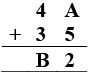Since we know that,
12 – 5 = 7 = A
So, B = 8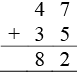Hence, A = 7 and B = 8

(ii)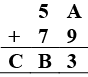Since we know that,
9 + 4 = 13,
∴ A = 4
B = 1 + 5 + 7 = 3
C = 1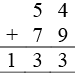Hence, A = 4, B = 3, C = 1

(iii)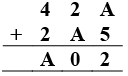Since we know that,
5 + 7 = 12, ∴ A = 7
1 + 2 + 7 = 10
1 + 4 + 2 = 7 = A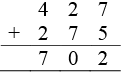Hence, A = 7

(iv)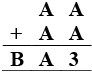Since we know that,
A = 4 or 9
A ≠ 4 as A + A = A, 4 + 4 ≠ 4
A = 9
B = 1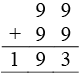Hence A = 9, B = 1

(v)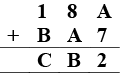Since we know that,
5 + 7 = 12
∴ A = 5
B = 4, C = 6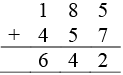Hence, A = 5, B = 4, C = 6

(vi)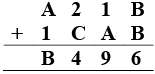Since we know that,
B = 3 or 8
If B = 8
1 + 1 + 7 = 9 then A = 7
C = 4 – 2 = 2
7 + 1 = 8 = B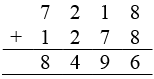Hence, A = 7, B = 8, C = 2

(vii)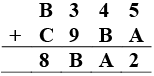Since we know that,
A = 7 (∵ 5 + 7 =12)
1 + 4 + 2 = 7(A)
∴ B = 2
1 + 2 + 5 = 8, C = 5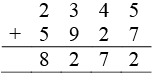Hence, A = 7, B = 2, C = 5

(viii)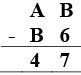Since we know that,
B – 6 = 7
B = 3
A – 1 – B = 4
A – 1 – 3 = 4, A = 4 + 4 = 8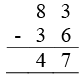Hence, A = 8, B = 3

(ix)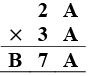Since we know that,
A = 1 or 6 or 5 as 1 × 1 = 1
or 6 × 6 = 6
or 5 × 5 = 5
Taking A = 5
B = 8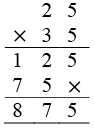Hence, A = 5, B = 8

(x)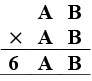Since we know that,
B × B = B
B = 1, 6, 5
If B = 5, and A = 2,
then 25 × 25 = 625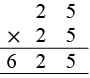Hence, A = 2 and B = 5

(xi)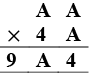Since we know that,
A = 2 or 8
Let A = 2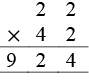Hence, A = 2

Q.2. Fill in the numbers from 1 to 6 (without repetition) so that each side of the magic triangle adds up to 12.Given:
Numbers 1 to 6 without repetition:
The sum from each side = 12
There can be much more solutions such as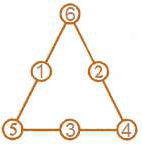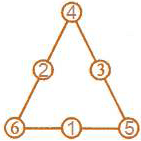Q.3. Complete the magic square given alongside using number 0, 1, 2, 3, ……., 15 (only once), so that sum along each row, column and diagonal is 30.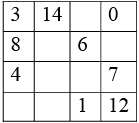Given:
In the magic square, the use of number 0, 1, 2, 3… 15
So, only once the sum along each row, column and diagonal is 30.
Some numbers are already filled.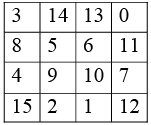3 + 8 + 4 = 15 + 15 = 30
3 + 12 = 5 + 10 = 30
3 + 14 + 0 = 17 + 13 = 30
0 + 6 = 9 + 15 = 30
0 + 7 + 12 = 19 + 11 =30
15 + 1 + 12 = 28 + 2 = 30
8 + 6 + 11 = 25 + 5 = 30
13 + 6 + 1 = 20 + 10 = 30
14 + 5 + 2 = 21 + 9 = 30

The document ML Aggarwal: Playing with Numbers - 2 Notes | Study ML Aggarwal Solution: Class 8 Math - Class 8 is a part of the Class 8 Course ML Aggarwal Solution: Class 8 Math.
All you need of Class 8 at this link: Class 8

## ML Aggarwal Solution: Class 8 Math

58 docs
 Use Code STAYHOME200 and get INR 200 additional OFF

## ML Aggarwal Solution: Class 8 Math

58 docs

Track your progress, build streaks, highlight & save important lessons and more!

,

,

,

,

,

,

,

,

,

,

,

,

,

,

,

,

,

,

,

,

,

;